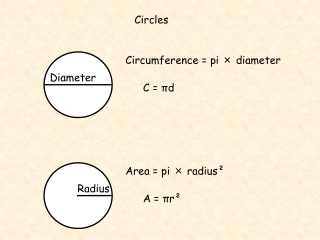DownloadDownload PresentationCircles

# Circles

Download Presentation## Circles

- - - - - - - - - - - - - - - - - - - - - - - - - - - E N D - - - - - - - - - - - - - - - - - - - - - - - - - - -
##### Presentation Transcript

1. Radius Circles Circumference = pi × diameter Diameter C = πd Area = pi × radius² A = πr²

2. The Circumference of a Circle Find the circumference of the following circles. 1 2 8 cm 9.5 cm C =d C =d C=  x 8 C = 25.1 cm (1 dp) C =d C=  x 9.5 C = 29.8 cm (1 dp)

3. The Circumference of a Circle Find the circumference of the following circles. 4 3 3 mm C =d 2.1 m C =2r C = 2 x  x 3 C = 18.8 mm(1 dp) C = 2r C = 2 x  x 2.1 C = 13.2 m(1 dp)

4. The Circumference of a Circle Find the perimeter of the following semi-circles. 1 2 8 cm 9.5 cm C =d Perimeter =½d + d = ½ x  x 9.5 + 9.5 = 24.4 cm (1 dp) Perimeter =½d + d = ½ x  x 8 + 8 = 20.6 cm (1 dp)

5. The Circumference of a Circle Find the perimeter of the ¼ and ¾ circles. 3 4 8.5 cm 6 cm C =d Perimeter =¾(2r) + 2r = ¾ x 2 x  x 8.5 + 2 x 8.5 = 57.1 cm (1 dp) Perimeter =¼(2r) + 2r = ¼ x 2 x  x 6 + 2 x 6 = 21.4 cm (1 dp)

6. C =d The Circumference of a Circle Find the diameter/radius of the following circles. 5 6 C = 25 cm find the diameter. C = 30 cm find the radius. 2r = 30 r = 30/(2) r = 4.8 cm (1 dp) d = 25 d = 25/ d = 8.0 cm (1 dp)

7. A =r2 The Area of a Circle Find the area of the following circles. 1 2 8 cm 9.5 cm A =r2 A =  x 82 A = 201.1 cm2 (1 dp) A =r2 A =  x 9.52 A = 283.5 cm2 (1 dp)

8. The Area of a Circle A =r2 Find the area of the following circles. 3 4 6 mm 2.4 m A =r2 A =  x 32 A = 28.3 mm2 (1 dp) A =r2 A =  x 1.22 A = 4.5 m2 (1 dp)

9. Find the area of the clock face and radar screen. A =r2 12 cm 60 cm A =r2 A =  x 122 A = 452.4 cm2 (1 dp) A =r2 A =  x 302 A = 2827 cm2 (nearest cm2)

10. A =r2 The Area of a Circle Find the area of the following semi-circles. 1 2 8 cm 9.5 cm A =½r2 = ½ x  x 4.752 = 35.4 cm2 (1 dp) A =½r2 = ½ x  x 42 = 25.1 cm2 (1 dp)

11. The Area of a Circle A =r2 Find the area of the ¼ and ¾ circles. 3 4 8.5 cm 6 cm A =¾r2 = ¾ x  x 8.52 = 170.2 cm2 (1 dp) A =¼r2 = ¼ x  x 62 = 28.3 cm2 (1 dp)

12. The Area of a Circle A =r2 Find the radius/diameter of the following circles. 6 5 A = 25 cm2 find the diameter. A = 30 cm2 find the radius. r2 = 25 r2 = 25/ r = (25/) r = 2.82 d = 2 x 2.82 = 5.6 cm 1dp r2 = 30 r2 = 30/ r = (30/) r = 3.1 cm 1dp